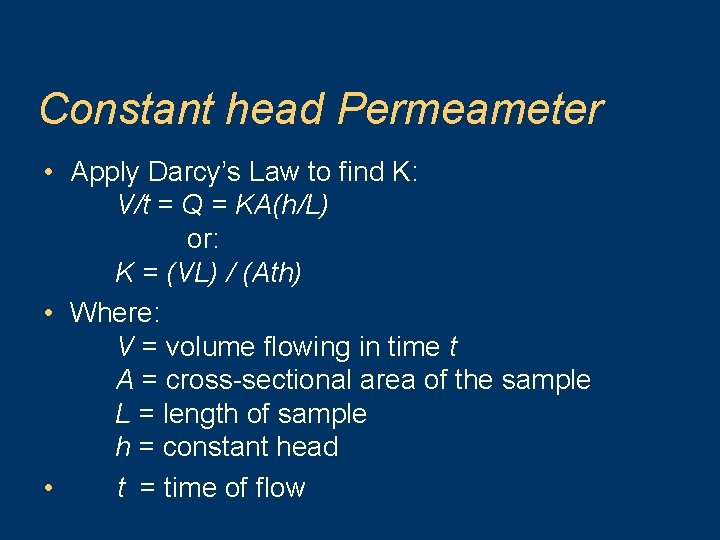# Darcys Law Philip B Bedient Civil and Environmental

• Slides: 24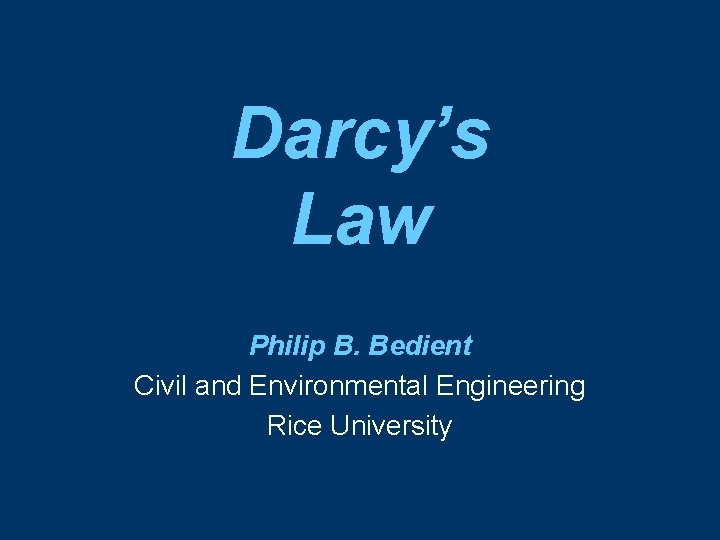Darcy’s Law Philip B. Bedient Civil and Environmental Engineering Rice University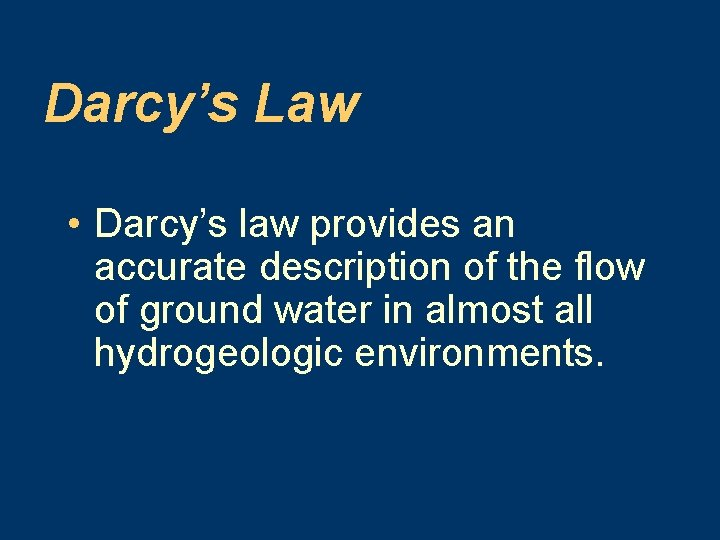Darcy’s Law • Darcy’s law provides an accurate description of the flow of ground water in almost all hydrogeologic environments.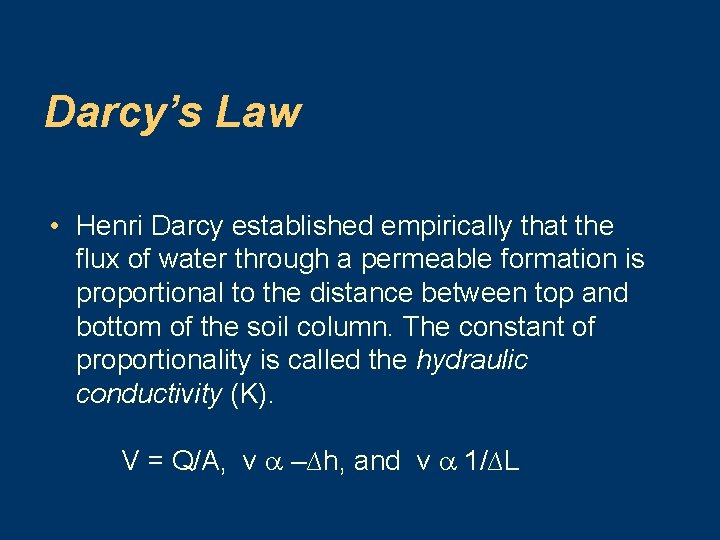Darcy’s Law • Henri Darcy established empirically that the flux of water through a permeable formation is proportional to the distance between top and bottom of the soil column. The constant of proportionality is called the hydraulic conductivity (K). V = Q/A, v –∆h, and v 1/∆L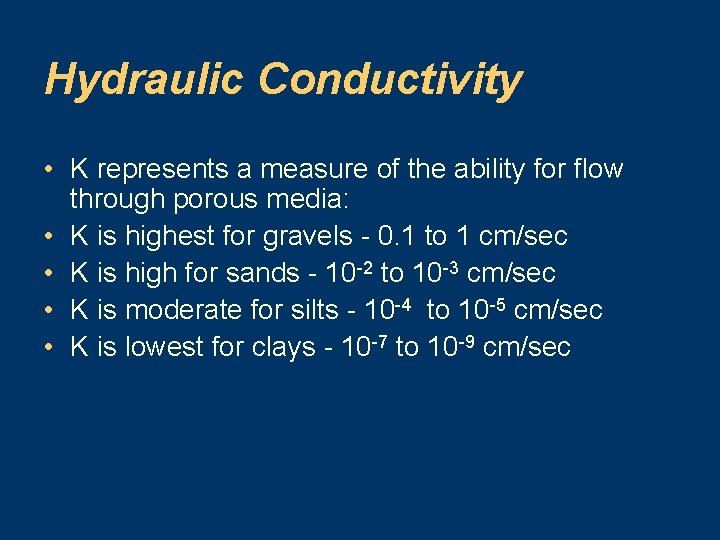Hydraulic Conductivity • K represents a measure of the ability for flow through porous media: • K is highest for gravels - 0. 1 to 1 cm/sec • K is high for sands - 10 -2 to 10 -3 cm/sec • K is moderate for silts - 10 -4 to 10 -5 cm/sec • K is lowest for clays - 10 -7 to 10 -9 cm/sec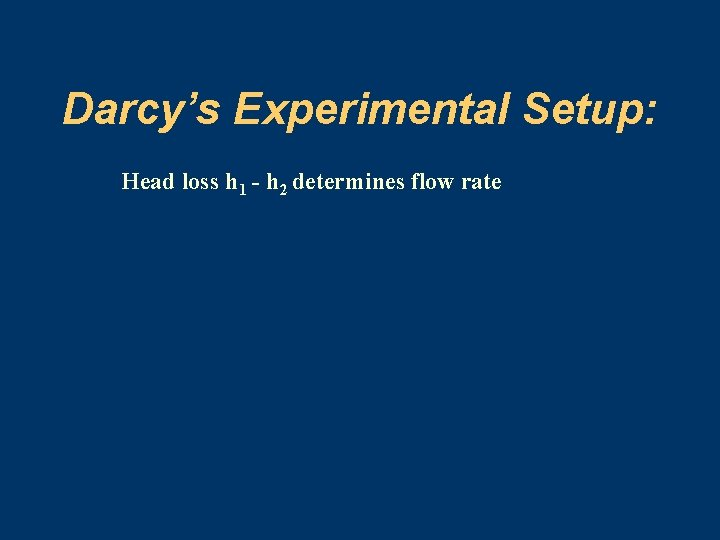Darcy’s Experimental Setup: Head loss h 1 - h 2 determines flow rate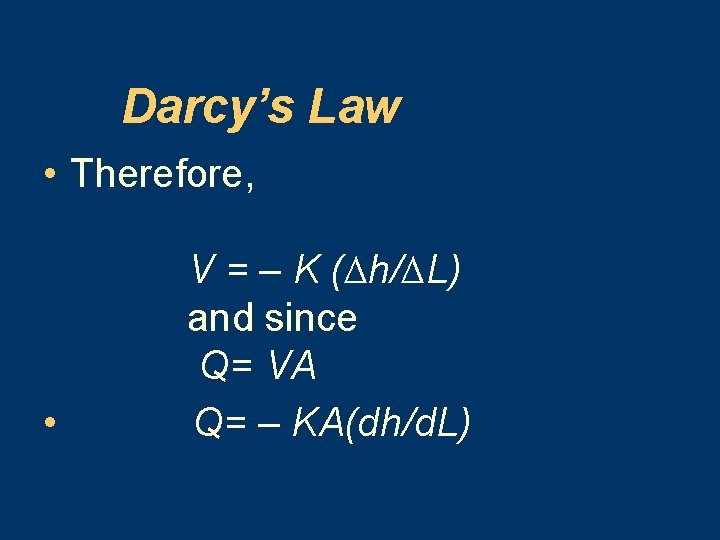Darcy’s Law • Therefore, • V = – K (∆h/∆L) and since Q= VA Q= – KA(dh/d. L)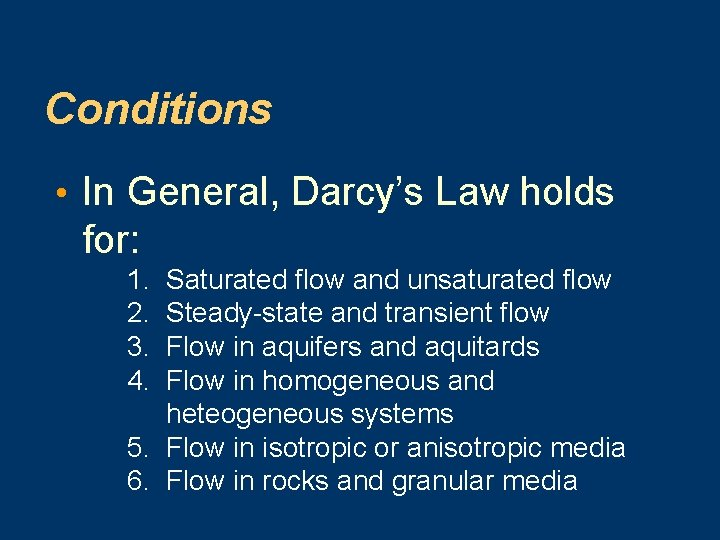Conditions • In General, Darcy’s Law holds for: 1. 2. 3. 4. Saturated flow and unsaturated flow Steady-state and transient flow Flow in aquifers and aquitards Flow in homogeneous and heteogeneous systems 5. Flow in isotropic or anisotropic media 6. Flow in rocks and granular mediaDarcy Velocity • V is the specific discharge (�Darcy velocity). • (–) indicates that V occurs in the direction of the decreasing head. • Specific discharge has units of velocity. • The specific discharge is a macroscopic concept, and is easily measured. It should be noted that Darcy’s velocity is different ….Darcy Velocity • . . . from the microscopic velocities associated with the actual paths if individual particles of water as they wind their way through the grains of sand. • The microscopic velocities are real, but are probably impossible to measure.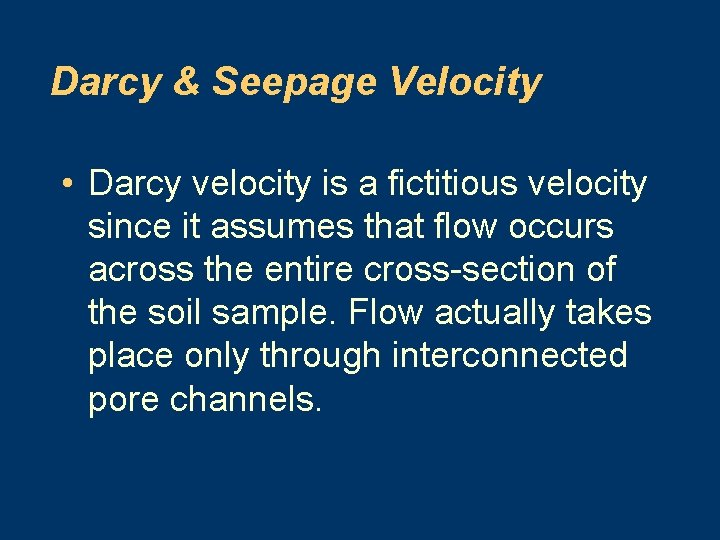Darcy & Seepage Velocity • Darcy velocity is a fictitious velocity since it assumes that flow occurs across the entire cross-section of the soil sample. Flow actually takes place only through interconnected pore channels.Darcy & Seepage Velocity • From the Continuity Eqn: • Q = A v. D = A V V s – Where: Q = flow rate A = cross-sectional area of material AV= area of voids Vs = seepage velocity v. D= Darcy velocityDarcy & Seepage Velocity • Therefore: VS = VD ( A/AV) • Multiplying both sides by the length of the medium (L) VS = VD ( AL / AVL ) = VD ( VT / VV ) • Where: VT = total volume VV = void volume • By Definition, Vv / VT = n, the soil porosity • Thus VS = VD/nEquations of Ground Water Flow • Description of ground water flow is based on: 1. Darcy’s Law 2. Continuity Equation - describes conservation of fluid mass during flow through a porous medium; results in a partial differential equation of flow.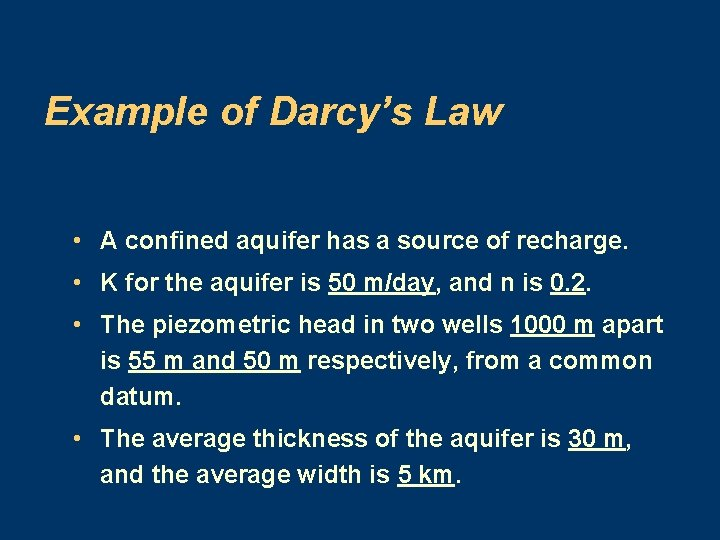Example of Darcy’s Law • A confined aquifer has a source of recharge. • K for the aquifer is 50 m/day, and n is 0. 2. • The piezometric head in two wells 1000 m apart is 55 m and 50 m respectively, from a common datum. • The average thickness of the aquifer is 30 m, and the average width is 5 km.Calculate: • a) the rate of flow through the aquifer • (b) the time of travel from the head of the aquifer to a point 4 km downstream • *assume no dispersion or diffusionThe solution • Cross-Sectional area= 30(5)(1000) = 15 x 104 m 2 • Hydraulic gradient = (55 -50)/1000 = 5 x 10 -3 • Rate of Flow for K = 50 m/day Q = (50 m/day) (75 x 101 m 2) = 37, 500 m 3/day • Darcy Velocity: V = Q/A = (37, 500 m 3/day) / (15 x 104 m 2) = 0. 25 m/dayAnd • Seepage Velocity: Vs = V/n = (0. 25) / (0. 2) = 1. 25 m/day (about 4. 1 ft/day) • Time to travel 4 km downstream: T = 4(1000 m) / (1. 25 m/day) = 3200 days or 8. 77 years • This example shows that water moves very slowly underground.Limitations of the Darcian Approach 1. For Reynold’s Number, Re, > 10 where the flow is turbulent, as in the immediate vicinity of pumped wells. 2. Where water flows through extremely fine-grained materials (colloidal clay)Darcy’s Law: Example 2 • A channel runs almost parallel to a river, and they are 2000 ft apart. • The water level in the river is at an elevation of 120 ft and 110 ft in the channel. • A pervious formation averaging 30 ft thick and with K of 0. 25 ft/hr joins them. • Determine the rate of seepage or flow from the river to the channel.Confined Aquifer Confining Layer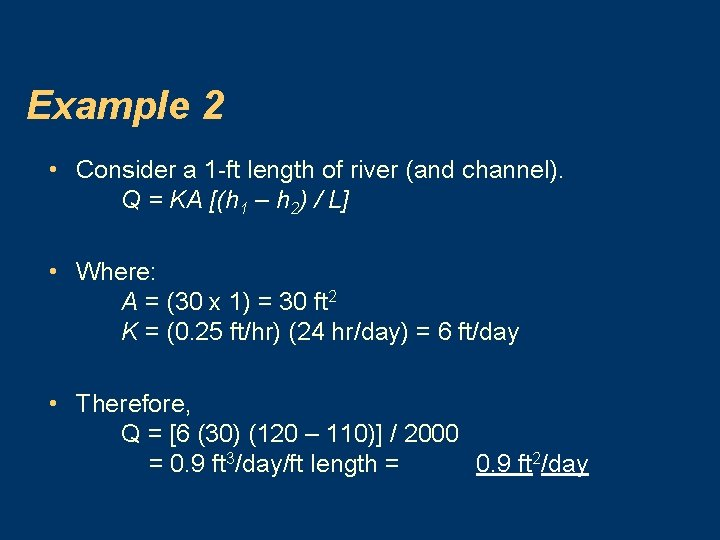Example 2 • Consider a 1 -ft length of river (and channel). Q = KA [(h 1 – h 2) / L] • Where: A = (30 x 1) = 30 ft 2 K = (0. 25 ft/hr) (24 hr/day) = 6 ft/day • Therefore, Q = [6 (30) (120 – 110)] / 2000 = 0. 9 ft 3/day/ft length = 0. 9 ft 2/day